Fitting power law data

Consider a random variable $x$ that takes values governed by a power law probability distribution, $p(x)=C x^{-\alpha}$.

First we generate some synthetic data with a power law distribution:

In :
alpha=2.5
xmin=1
n=1000
xr=xmin*rand(n)**(-1/(alpha-1))

[This uses the so-called "transformation method": if $r$ is a random real number uniformly distributed in the range $0 < r \le 1$, then it is straightforward to show that $x = x_{\rm min}r^{−1/(\alpha−1)}$ is a random power-law-distributed real number in the range $x_{\rm min} \le x < \infty$, with probability distribution governed by exponent $\alpha$, i.e., $p(x)=C x^{-\alpha}$.]

Here is a histogram of the above 1000 data points, with number counts in bins of linear size .1:

In :
figure(figsize=(6,5))
yh,bh,p=hist(xr,bins=arange(xmin,1000,.1),log=True,histtype='step')
xlabel('x')
ylabel('# occurrences in bin of size .1')
ylim(.5,300),xlim(1,200)
xscale('log');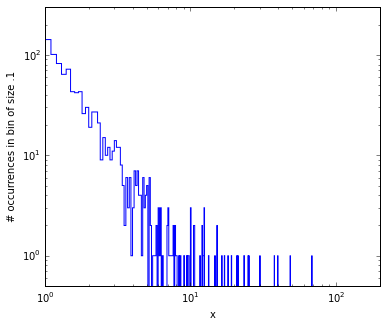An obvious way to try to fit a power law to this data is to use the linregress() function, as described in lec19, applied to the log-log data (where we're careful only to use the bins with non-zero counts):

In :
from scipy.stats import linregress
slope, intercept, r_value, p_value, std_err = linregress(log(bh[yh>0]),log(yh[yh>0]))
print slope,intercept

figure(figsize=(6,5))
yh,bh,p=hist(xr,bins=arange(xmin,1000,.1),log=True,histtype='step')
xlabel('x')
ylabel('# occurrences in bin of size .1')
ylim(.5,300),xlim(1,200)
xscale('log')

xl=arange(1,200,.1)
plot(xl,exp(intercept)*xl**(slope),'k-',linewidth=2,label='$\\alpha={:.2f}$'.format(-slope))

legend();
-1.27505386327 3.51358457684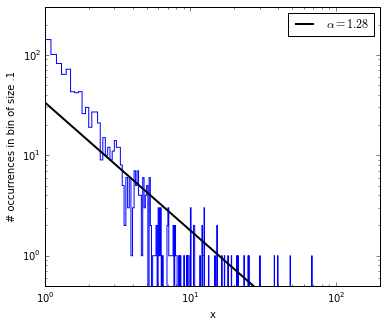This is not very successful, given that the data was generated with $\alpha=2.5$. The problem appears to be that the number counts are small out in the tail so they drag the best fit to the wrong slope. Suppose we instead fit just the first $x_{\rm max}$ points, for $x_{\rm max}$ in (64,32,16,8,4,2):

In :
figure(figsize=(6,5))
yh,bh,p=hist(xr,bins=arange(xmin,1000,.1),log=True,histtype='step')
xlabel('x')
ylabel('# occurrences in bin of size .1')
ylim(.5,300),xlim(1,200)
xscale('log')

slope, intercept = linregress(log(bh[yh>0]),log(yh[yh>0]))[:2]
xl=arange(1,200,.1)
plot(xl,exp(intercept)*xl**(slope),'k-',linewidth=2,
label='$\\alpha={:.2f}$ (all)'.format(-slope))

for xmax in (64,32,16,8,4,2):
retain=logical_and(yh>0,bh[:-1]<=xmax)
slope,intercept = linregress(log(bh[retain]),log(yh[retain]))[:2]
xl=arange(1,xmax+1,xmax-1)
plot(xl,exp(intercept)*xl**(slope),linewidth=1,
label='$\\alpha={:.2f}$ ({})'.format(-slope,xmax));
legend();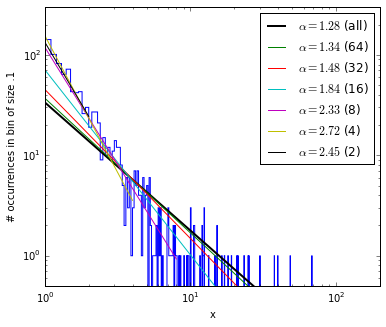This gives a number of different slopes, from too small to too large, with no principled method for deciding where to apply the cut. A more principled method (derived) below, suggests instead to use all data points $x_i$ above some $x_{\rm min}$ (in this case $x_{\rm min}=1$), suppose that's $n$ data points, then calculate: $$\alpha = 1 + {n\over \sum_{i=1}^n \ln {x_i\over x_{\rm min}}}$$ with the result:

In :
alpha=1+n/sum(log(xr))
sigma=(alpha-1)/sqrt(n)
print 'alpha=',round(alpha,3),', with stderr sigma=',round(sigma,3)
alpha= 2.52 , with stderr sigma= 0.048

How does this more principled method work?

First consider the normalization constant $C$ in the probability $p(x)=C x^{-\alpha}$, determined by

$$1=\int_{x_{\rm min}}^\infty p(x){\rm d}x=\int_{x_{\rm min}}^\infty C x^{-\alpha} ={C\over 1-\alpha}x^{-\alpha+1}\Big|_{x_{\rm min}}^\infty={C\over \alpha-1}x_{\rm min}^{-\alpha+1}$$

(where $\alpha>1$ is assumed so that the integral converges). The result is

$$C=(\alpha-1)x_{\rm min}^{\alpha-1}\ .$$

Now consider the probability of the data. Given a set of $n$ values $x_i$, the probability $P(x|\alpha)$ that they were generated by the $p(x)$ distribution (likelihood of the dataset) is the product of the probabilities:

$$P(x|\alpha)=p(x_1)\cdots p(x_n)=\prod_{i=1}^np(x_i)=\prod_{i=1}^nC x_i^{-\alpha} \ .$$

The $\alpha$ that provides the best fit for the data is given by maximizing $P(\alpha|x)$, in turn given by Bayes theorem as

$$P(\alpha|x)={P(x|\alpha)P(\alpha)\over P(x)}\ .$$

But $P(x)$ is fixed for a given set of data $x$, and for no prior knowledge of $\alpha$ it is natural to assume $P(\alpha)$ if flat (i.e., with no $\alpha$ dependence). Then $P(\alpha|x)$ is just proportional to $P(x|\alpha)$ and $\alpha$ is chosen to maximize the above likelihood $P(x|\alpha)$ of the dataset.

Since the logarithm is an increasing function, it is equivalent (and slightly more convenient) to maximize the log of the function :

$${\cal L}(\alpha)=\ln P(x|\alpha) = \sum_{i=1}^n (\ln(C) + \ln x_i^{-\alpha})= n\ln C-\alpha\sum_{i=1}^n \ln x_i = n\ln \bigl[(\alpha-1)x_{\rm min}^{\alpha-1}\bigr] -\alpha\sum_{i=1}^n \ln x_i\ .$$

Setting to zero the derivative with respect to $\alpha$,

$$0={d\over d\alpha}{\cal L}(\alpha)= n{1\over \alpha-1} -\sum_{i=1}^n \ln {x_i\over x_{\rm min}}$$

gives the value of the exponent $\alpha$ that maximizes the likelihood of the data (for the assumed $p(x)=C x^{-\alpha}$):

$$\alpha = 1 + {n\over \sum_{i=1}^n \ln {x_i\over x_{\rm min}}}$$

With a bit more effort (see, e.g., eqns B7-B12 from appendix B here), the expected error (stdev) of the estimation of the exponent $\alpha$ can be shown to be given by the simple formula

$$\sigma={(\alpha -1)\over\sqrt{n}}$$

(which decreases, as expected, as the inverse square root of the number of data points).

Finally, here is the data again showing the relation to the best fit power law distribution:

In :
C=(alpha-1)*xmin**(alpha-1)

figure(figsize=(6,5))
yh,bh,p=hist(xr,bins=arange(xmin,1000,.1),log=True,histtype='step')
xlabel('x')
ylabel('# occurrences in bin of size .1')
ylim(.5,300),xlim(1,200)
xscale('log')

xl=arange(1,200,.1)
plot(xl,(.1*n)*C*xl**(-alpha),'k-',linewidth=2,label='$\\alpha={:.2f}$'.format(alpha))

legend();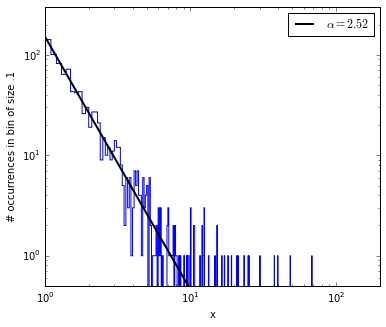Finally, here is the same thing with n= 1 million data points:

In :
alpha=2.5
xmin=1
n=1000000
xr=xmin*rand(n)**(-1/(alpha-1))
In :
alpha=1+n/sum(log(xr))
sigma=(alpha-1)/sqrt(n)
print 'alpha=',round(alpha,4),', with stderr sigma=',round(sigma,4)
C=(alpha-1)*xmin**(alpha-1)

figure(figsize=(6,5))
yh,bh,p=hist(xr,bins=arange(xmin,20000,.1),log=True,histtype='step')
xlabel('x')
ylabel('# occurrences in bin of size .1')
ylim(.5,150000),xlim(1,20000)
xscale('log')
xl=arange(1,200,.1)
plot(xl,(.1*n)*C*xl**(-alpha),'r-',linewidth=2,label='$\\alpha={:.4f}$'.format(alpha))
title('n={:,} data points'.format(n))
legend();
alpha= 2.5004 , with stderr sigma= 0.0015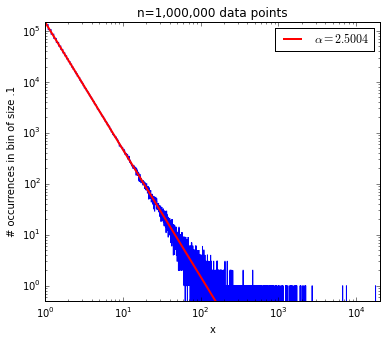In [ ]: